### Global curvature, ideal knots and models of DNA packing

 Global curvature characterizes knot tightness.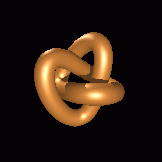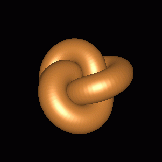#### Overview and acknowledgements

During a lunch-time discussion on the ideal shapes of knots and such, the concept of the "global curvature function" of a space curve was born. This function is related to, but distinct from, standard local curvature, and is connected to various physically appealing properties of a curve. Global curvature provides a concise characterization of curve thickness, and of certain ideal shapes of knots as have been investigated within the context of DNA. Moreover, global curvature is connected to the writhing number of a space curve and has applications in the study of self-contact and packing problems for rods. Some of the figures here are based on numerical data from

Katritch, V., Bednar, J., Michoud, D., Scharein, R.G., Dubochet, J. & Stasiak, A. (1996) Nature 384, 142-145.

Katritch, V., Olson, W.K., Pieranski, P., Dubochet, J. & Stasiak, A. (1997) Nature 388, 148-151.

It is a pleasure to thank A. Stasiak, V. Katritch and P. Pieranski for making their knot data available.

#### Related articles

O. Gonzalez & R. de la Llave, "Existence of ideal knots," Journal of Knot Theory and Its Ramifications 12 (2003) 123-133.

J.R. Banavar, O. Gonzalez, J.H. Maddocks & A. Maritan, ``Self-interactions of strands and sheets,'' Journal of Statistical Physics 110 (2003) 35-50.

O. Gonzalez, J.H. Maddocks & J. Smutny, ``Curves, circles and spheres,'' in Physical Knots: Knotting, Linking and Folding Geometric Objects in R^3, J.A. Calvo, K.C. Millett & E.J. Rawdon, Eds., Contemporary Mathematics 304 (2002) 195-215.

O. Gonzalez, J.H. Maddocks, F. Schuricht & H. von der Mosel, ``Global curvature and self-contact of nonlinearly elastic curves and rods,'' Calculus of Variations and Partial Differential Equations, 14 (2002) 29-68.

O. Gonzalez & J.H. Maddocks, "Global Curvature, Thickness and the Ideal Shapes of Knots," The Proceedings of the National Academy of Sciences, USA, 96 (1999) 4769-4773.

### Motivation

Any smooth, non-self-intersecting curve can be thickened into a smooth, non-self-intersecting tube of constant radius centered on the curve, as illustrated below.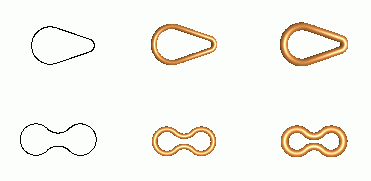If the curve is a straight line there is no upper bound on the tube radius, but for non-straight curves there is a critical radius above which the tube either ceases to be smooth or exhibits self-contact. This critical radius is an intrinsic property of the curve called its thickness or normal injectivity radius. Of the two examples shown above, the first has a critical radius determined by smoothness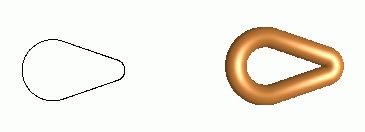and the second has a critical radius determined by self-contact.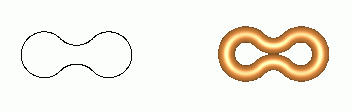If one considers the class of smooth, non-self-intersecting closed curves of a prescribed knot type and unit length, one may ask which curve in this class is the thickest. The thickness of such a curve is an intrinsic property of the knot, and the curve itself provides a certain ideal shape or representation of the knot type. For example, the two curves shown below are of the same knot type (K31), but the second is substantially thicker than the first. In fact, the second is considered to be of maximum thickness for its knot type -- it is considered to be an ideal shape for the K31 knot.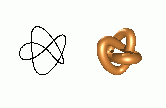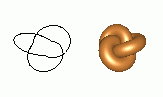Approximations of ideal shapes in above sense have been found via a series of computer experiments by Katritch and coworkers. These shapes were seen to have intriguing physical features, and even a correspondence to time-averaged shapes of knotted DNA molecules in solution.

So how does one mathematically define curve thickness and the ideal shape of a knot? What are necessary and sufficient conditions for a knotted curve to be ideal? Answering these questions has led to a new geometrical quantity for space curves: the global radius of curvature function. This function provides a simple characterization of curve thickness, and provides an elementary necessary condition that any ideal shape of a knotted curve must satisfy. Moreover, global radius of curvature is connected to the writhing number of a space curve and has applications in the study of self-contact problems for rods.

### Definition of global curvature

Our definition of global radius of curvature is based on the elementary facts that any three non-collinear points x, y and z in three-dimensional space define a unique circle (the circumcircle), and the radius of this circle (the circumradius) can be written as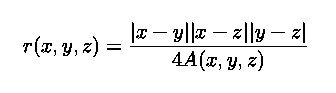where A(x,y,z) is the area of the triangle with vertices x, y and z, |x-y| is the Euclidean distance between the points x and y, and so on. When the points x, y and z are distinct, but collinear, the circumcircle degenerates into a straight line and we assign a value of infinity to r(x,y,z).

When x, y and z are points on a simple, smooth curve C, the domain of the function r(x,y,z) can be extended by continuous limits to all points on C.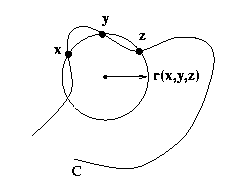For example, the limit of r(x,y,z) as y,z approach x along C is just the standard local radius of curvature at x, and the limit circumcircle is just the osculating circle to C at x. If one holds x and y fixed, and takes the limit as z approaches y along C, then the limiting value of r(x,y,z) is the radius of that circle which passes through x and is tangent to C at y.

Given a simple, smooth curve C we define the global radius of curvature at each point x by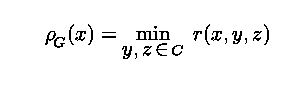which can actually be shown to be a continuous function of x on C. Global radius of curvature can be interpreted as a generalization, indeed a globalization, of the standard local radius of curvature. In fact, since the points y=x and z=x are competitors in the minimization, global radius of curvature is bounded by local radius of curvature, that is,The figure below shows plots of global (black) and local (grey) radius of curvature versus arclength for some example space curves C. The space curves are represented by their critical tubes, which are colored by global radius of curvature. The blue regions are where global radius of curvature is minimal.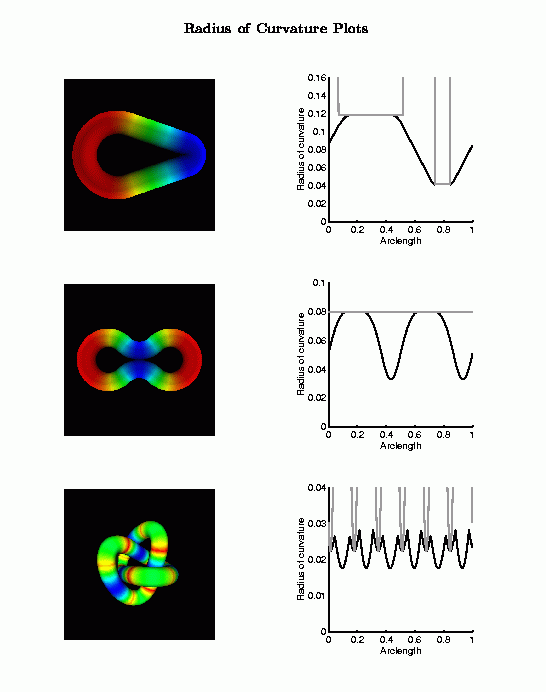### Applications of global curvature

A particularly interesting quantity for a simple, smooth curve C is the minimum value of global radius of curvature, namelywhich is just the minimum value of the circumradius function r(x,y,z) over all triplets of points on C. This quantity has many physically appealing interpretations and applications:

• Any spherical shell of radius less than Delta[C] cannot intersect C in three or more points (counting tangency points twice). In effect, a billiard ball of radius less than Delta[C] cannot find a stable resting place in C, for there is always enough room for it to pass through the curve, as illustrated in the figure below.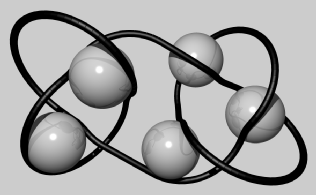• Delta[C] is the thickness of C in the sense that it is the radius of the thickest smooth tube that may be centered on C, as illustrated in the figure below.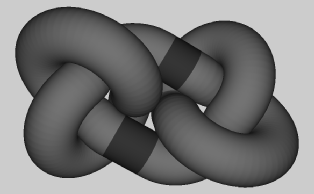### Ideal knots

#### definition

We can now use rhoG(x) and Delta[C] to define and characterize ideal shapes of knots. Let K denote the set of smooth, non-self-intersecting closed curves of a prescribed knot type and length. Then a curve C* in K is ideal if and only if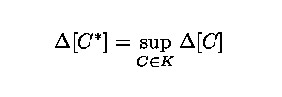That is, among all curves in K, an ideal shape C* has maximal thickness. This definition corresponds precisely to the intuitive notion of the thickest tube of fixed length that can be tied into a given knot.

#### a theorem

So what are the properties of curves C* that maximize Delta[C]? That is, what characterizes an ideal shape? Our study of this question led to the following theorem. Let C* be a curve in K with arclength parameter s in [0,L], and let J* be that subset of [0,L] for which the standard local curvature vanishes, that is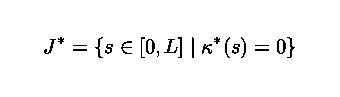Then C* can be ideal only if there is a constant a>0 such that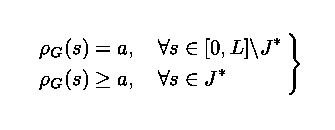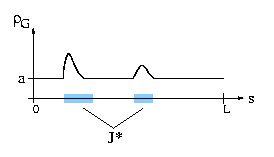This constant a>0 is actually the thickness of the ideal shape -- it is the radius of the thickest tube of fixed length that can be tied into the given knot. While we have defined everything here for smooth curves, there is a straightforward extension to discrete (piecewise linear) curves.

#### Yes, but is it sharp?

We tested our theorem on ideal shapes previously computed by A. Stasiak, V. Katritch and P. Pieranski. They had computed ideal shapes using hueristic, Monte Carlo type algorithms, and we had two main questions:

• First, would their data satisfy our necessary condition?

• Second, how sharp was it? Was the inequality "rhoG > a" a real possibility, or was it just a weakness in our arguments?
Our tests (along with some other neat data) are summarized in the following figures.

Results for non-ideal and ideal 3_1 knot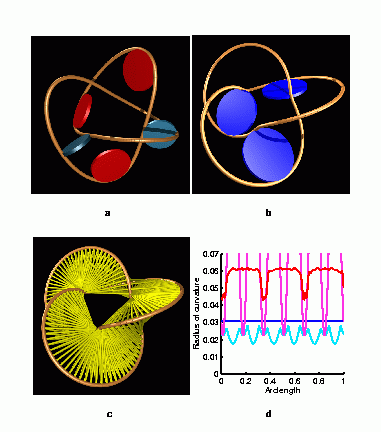• Frame a
• Generic knot shape produced using a simple parametric representation. The red discs indicate points where rhoG can be associated with the local radius of curvature, and blue discs indicate points where rhoG can be associated with a distance of closest approach.
• Frame b
• Numerically computed ideal shape. Here rhoG corresponds to a distance of closest approach at all points.
• Frame c
• Same shape as in b , but with a different visualization of the global radius of curvature. Each of the several spokes emanating from a point on the curve represents the diameter of a disc that realizes rhoG at that point.
• Frame d
• Global and local radius of curvature plots for the shapes in a and b .
• Light blue and light red curves are global and local radius of curvature for the non-ideal shape a .

• Dark blue and dark red curves are global and local radius of curvature for the ideal shape b .
(The light red curve is nearly periodic but its upper limits are not contained within the plot range.)
• Details on Ideal Shape
• 160 points nearly uniformly spaced in arclength
• computed using a Metropolis Monte Carlo procedure (Katritch et al.)
• variation in rhoG: 0.1 PERCENT
• our necessary condition is satisfied!
Results for ideal composite 3_1#3_1 knot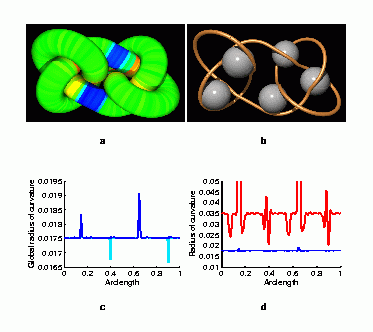• Frame a
• The tube shown here has a radius of Delta[C] where C is the centerline of the numerically computed ideal shape. The tube is colored by local curvature, where blue indicates near-zero values (straight portions).
• Frame b
• The sphere interpretation of Delta[C]. Any spherical shell of radius less than Delta[C] cannot intersect C in three or more points (counting tangency points twice). The spheres shown here have a radius of Delta[C].
• Frame c
• Global radius of curvature plot for shape in a . The light blue curve (partially obscured) corresponds to raw data from Katritch et al., and the dark blue curve corresponds to a corrected shape.
• Frame d
• Comparison of global radius of curvature (blue) and local radius of curvature (red) for the corrected shape.
• Details on Ideal Shape
• 286 points nearly uniformly spaced in arclength
• computed using a Metropolis Monte Carlo procedure (Katritch et al.)
• original data (light blue) was slightly non-ideal
• corrected data (dark blue) yielded ideal
• variation in rhoG (excluding two upward spike regions): 0.4 PERCENT
• our necessary condition is satisfied, and rhoG > a seems to be real!

### Self-contact problems

(new developments coming soon!)

### Optimal packing problems

(new developments coming soon!)